# 【图像缩放】双立方（三次）卷积插值

• 最邻近插值

• 双线性插值

• 双立方（三次卷积）插值

## 为何要进行双立方插值

• 对图像进行插值的目的是为了获取缩小或放大后的图片

• 常用的插值算法中，双立方插值效果最好

• 本文中介绍双立方插值的一些数学理论以及实现

双立方三次卷积只是这个插值算法的两种不同叫法而已，可以自行推导，会发现最终可以将求值转化为卷积公式

## 数学理论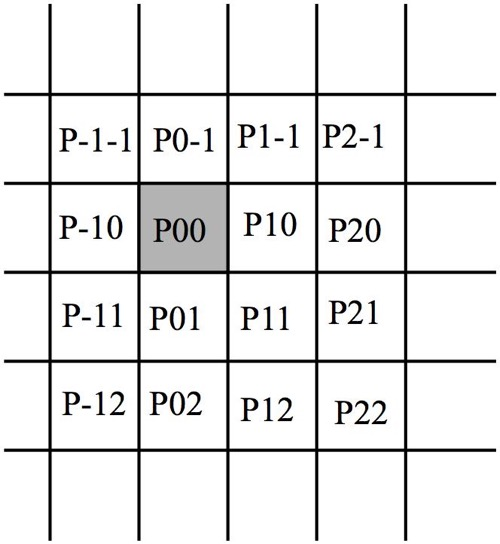• 其中P00代表目标插值图中的某像素点(x, y)在原图中最接近的映射点

• 譬如映射到原图中的坐标为(1.1, 1.1)，那么P00就是(1, 1)
• 而最终插值后的图像中的(x, y)处的值即为以上16个像素点的权重卷积之和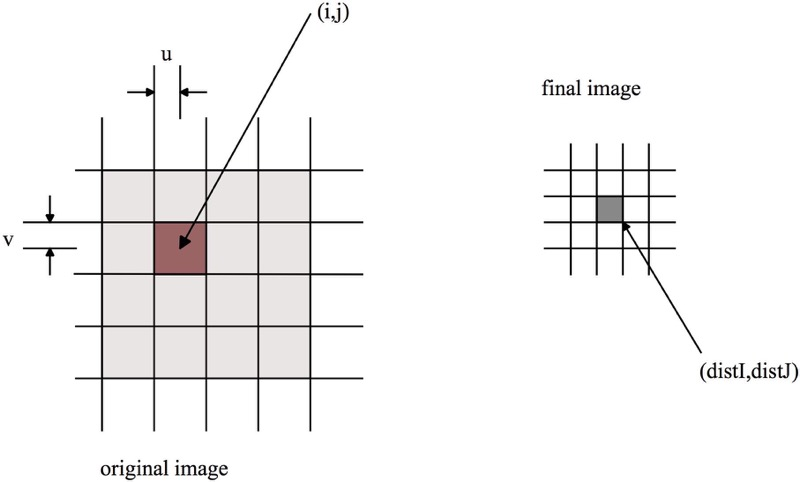• 譬如计算插值图中(distI, distJ)处像素的值

• 首先计算它映射到原图中的坐标(i + v, j + u)

• 也就是说，卷积计算时，p00点对应(i, j)坐标

• 最终，插值后的图(distI, distJ)坐标点对应的值是原图中(i, j)邻近16个像素点的权重卷积之和

• i, j的范围是[i - 1, i + 2][j - 1, j + 2]

### 卷积公式

• 设采样公式为S(x)

• 原图中每一个(i, j)坐标点的值得表达式为f(i, j)

• 插值后对应坐标的值为F(i + v, j + u)（这个值会作为(distI, distJ)坐标点的值）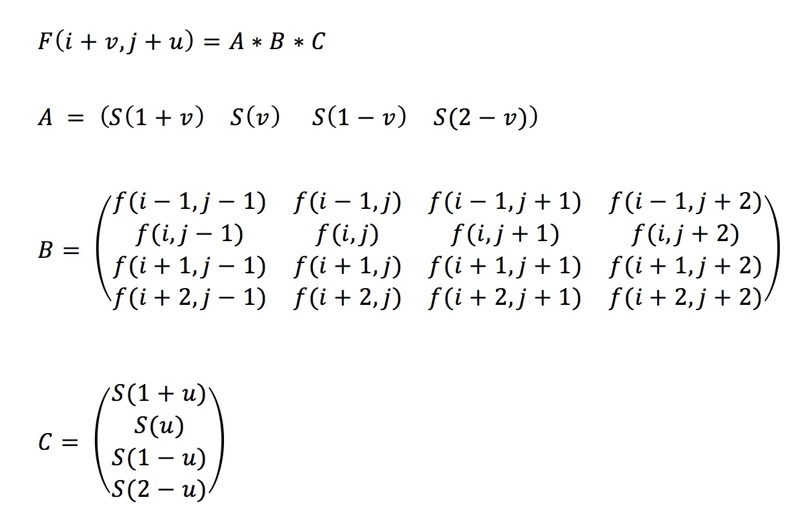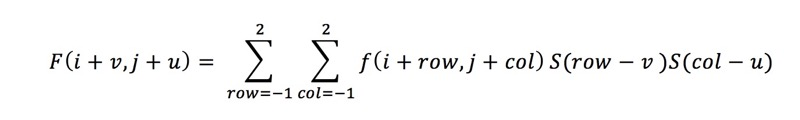### 采样公式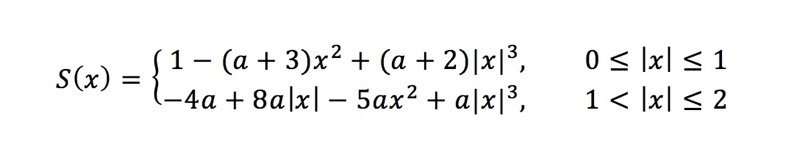• S(0) = 1

• S(n) = 0(当n为整数时)

• 当x超出范围时，S(x)为0

• a取不同值时可以用来逼近不同的样条函数（常用值-0.5, -0.75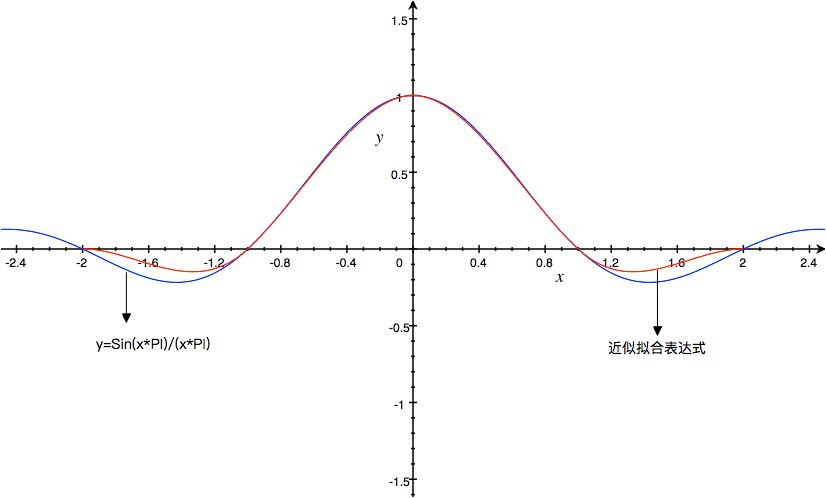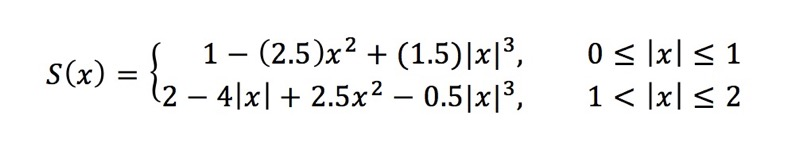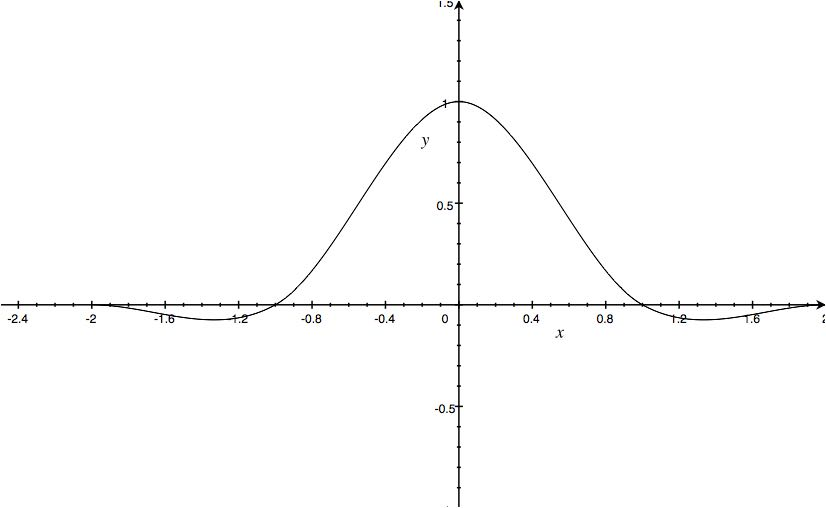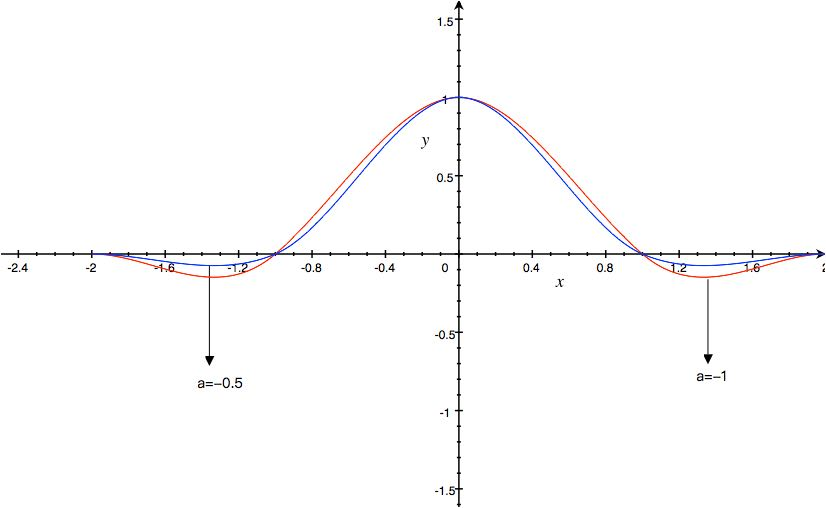### 关于网上的一些推导公式奇怪实现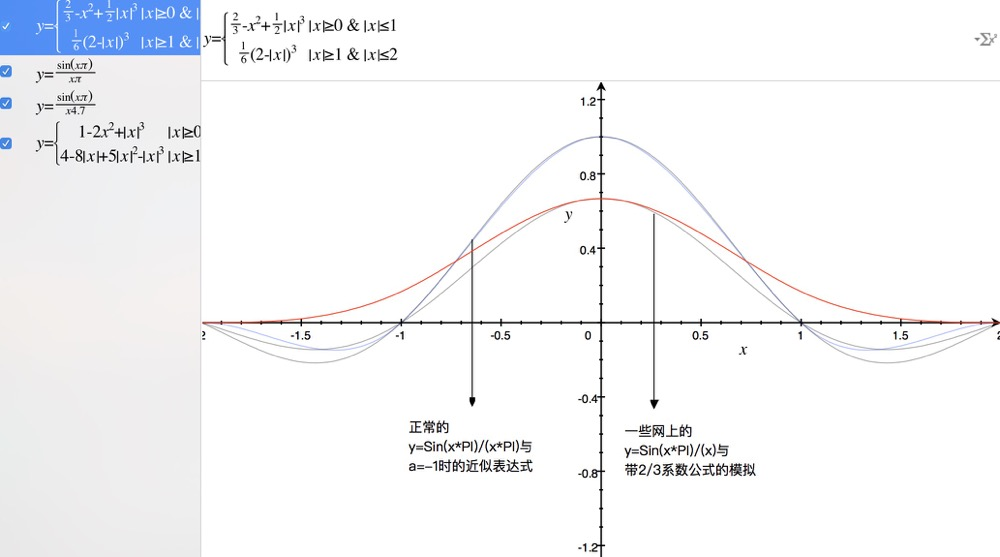## 代码实现

/**
* 采样公式的常数A取值,调整锐化与模糊
* -0.5 三次Hermite样条
* -0.75 常用值之一
* -1 逼近y = sin(x*PI)/(x*PI)
* -2 常用值之一
*/
const A = -0.5;

function interpolationCalculate(x) {
const absX = x > 0 ? x : -x;
const x2 = x * x;
const x3 = absX * x2;

if (absX <= 1) {
return 1 - (A + 3) * x2 + (A + 2) * x3;
} else if (absX <= 2) {
return -4 * A + 8 * A * absX - 5 * A * x2 + A * x3;
}
return 0;
}

/**
* 采样公式的常数A取值,调整锐化与模糊
* -0.5 三次Hermite样条
* -0.75 常用值之一
* -1 逼近y = sin(x*PI)/(x*PI)
* -2 常用值之一
*/
const A = -1;

function interpolationCalculate(x) {
const absX = x >= 0 ? x : -x;
const x2 = x * x;
const x3 = absX * x2;

if (absX <= 1) {
return 1 - (A + 3) * x2 + (A + 2) * x3;
} else if (absX <= 2) {
return -4 * A + 8 * A * absX - 5 * A * x2 + A * x3;
}

return 0;
}

function getPixelValue(pixelValue) {
let newPixelValue = pixelValue;

newPixelValue = Math.min(255, newPixelValue);
newPixelValue = Math.max(0, newPixelValue);

return newPixelValue;
}

/**
* 获取某行某列的像素对于的rgba值
* @param {Object} data 图像数据
* @param {Number} srcWidth 宽度
* @param {Number} srcHeight 高度
* @param {Number} row 目标像素的行
* @param {Number} col 目标像素的列
*/
function getRGBAValue(data, srcWidth, srcHeight, row, col) {
let newRow = row;
let newCol = col;

if (newRow >= srcHeight) {
newRow = srcHeight - 1;
} else if (newRow < 0) {
newRow = 0;
}

if (newCol >= srcWidth) {
newCol = srcWidth - 1;
} else if (newCol < 0) {
newCol = 0;
}

let newIndex = (newRow * srcWidth) + newCol;

newIndex *= 4;

return [
data[newIndex + 0],
data[newIndex + 1],
data[newIndex + 2],
data[newIndex + 3],
];
}

function scale(data, width, height, newData, newWidth, newHeight) {
const dstData = newData;

// 计算压缩后的缩放比
const scaleW = newWidth / width;
const scaleH = newHeight / height;

const filter = (dstCol, dstRow) => {
// 源图像中的坐标（可能是一个浮点）
const srcCol = Math.min(width - 1, dstCol / scaleW);
const srcRow = Math.min(height - 1, dstRow / scaleH);
const intCol = Math.floor(srcCol);
const intRow = Math.floor(srcRow);
// 计算u和v
const u = srcCol - intCol;
const v = srcRow - intRow;

// 真实的index，因为数组是一维的
let dstI = (dstRow * newWidth) + dstCol;

dstI *= 4;

// 存储灰度值的权重卷积和
const rgbaData = [0, 0, 0, 0];
// 根据数学推导，16个点的f1*f2加起来是趋近于1的（可能会有浮点误差）
// 因此就不再单独先加权值，再除了
// 16个邻近点
for (let m = -1; m <= 2; m += 1) {
for (let n = -1; n <= 2; n += 1) {
const rgba = getRGBAValue(
data,
width,
height,
intRow + m,
intCol + n,
);
// 一定要正确区分 m,n和u,v对应的关系，否则会造成图像严重偏差（譬如出现噪点等）
// F(row + m, col + n)S(m - v)S(n - u)
const f1 = interpolationCalculate(m - v);
const f2 = interpolationCalculate(n - u);
const weight = f1 * f2;

}
}

};

// 区块
for (let col = 0; col < newWidth; col += 1) {
for (let row = 0; row < newHeight; row += 1) {
filter(col, row);
}
}
}

export default function bicubicInterpolation(imgData, newImgData) {
scale(imgData.data,
imgData.width,
imgData.height,
newImgData.data,
newImgData.width,
newImgData.height);

return newImgData;
}

## 运行效果## 开源项目

https://github.com/dailc/image-process

## 附录

### 参考资料

posted @ 2017-11-02 20:12 撒网要见鱼 阅读(...) 评论(...) 编辑 收藏